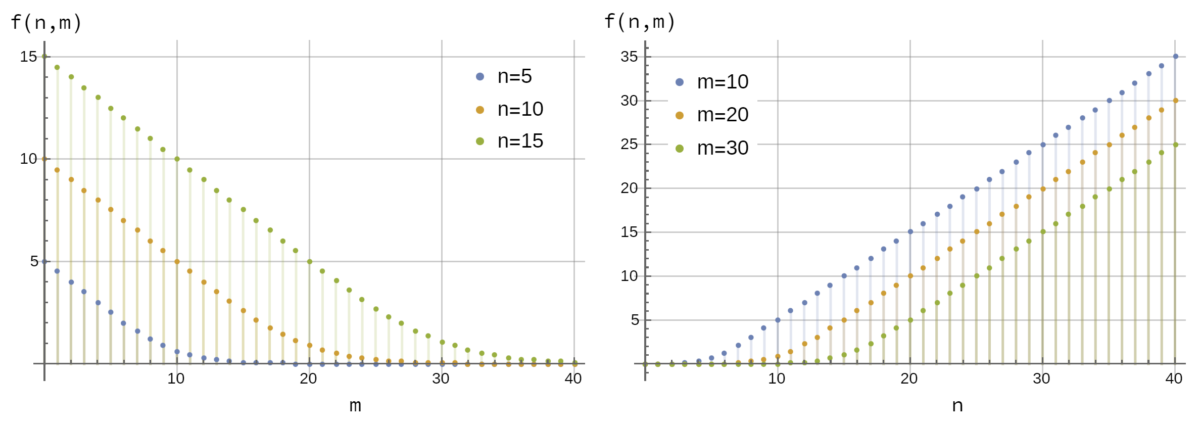# Squid gameThis week’s Riddler Classic is Squid Game-themed!

There are 16 competitors who must cross a bridge made up of 18 pairs of separated glass squares. Here is what the bridge looks like from above:To cross the bridge, each competitor jumps from one pair of squares to the next. However, they must choose one of the two squares in a pair to land on. Within each pair, one square is made of tempered glass, while the other is made of normal glass. If you jump onto tempered glass, all is well, and you can continue on to the next pair of squares. But if you jump onto normal glass, it will break, and you will be eliminated from the competition.

The competitors have no knowledge of which square within each pair is made of tempered glass. The only way to figure it out is to take a leap of faith and jump onto a square. Once a pair is revealed — either when someone lands on a tempered square or a normal square — all remaining competitors take notice and will choose the tempered glass when they arrive at that pair.

On average, how many of the 16 competitors will make it across the bridge?

Here is my solution.
[Show Solution]

And here is a much better solution!
[Show Solution]

## 4 thoughts on “Squid game”

1.Guy D. Moore says:

Hi Laurent,
A nice solution, just two quick comments.
You can make the recursion tighter by considering a single jump.
If there are n competitors and m bridge segments, and the next competitor jumps, either
s/he survives, leaving n competitors and m-1 segments, or
s/he dies, leaving n-1 and m-1: each has equal probability, so
f(n,m) = ( f(n,m-1) + f(n-1,m-1) )/2

The second comment is that the approximate solution is exact for n >= m;
each segment kills on average 1/2 competitor, so f(n,m) = n-m/2.
If n is almost m, you can find the (small) chance that the bridge “would have killed” n+1, n+2, …
and add the number of competitors who weren’t killed because they weren’t there to kill;
for the stated problem, there is a 1/2^18 chance that the bridge “could have killed” 18,
and an 18/2^18 chance that it “could have killed” 17, so the average number to survive is
7 + (18-16) * 1/2^18 + (17-16) * 18/2^18 = 7 + 5/2^16 which is the answer you find.

1.MarkS says:

Nice. That gives me enough hints for a closed-form solution. Let P be the number of players and S the number of segments (because n and m are too hard for me to remember which is which!). Suppose, at the end of the competition, every segment has been hit by at least one player. (If this is not the case, then no player has made it across.) Suppose B segments broke, and S-B did not. The probability of this outcome is C(S,B)/2^S, where C(S,B)=S!/(B!(S-B)!). Each broken segment eliminated exactly one player, so the number of players who made it across is P-B. To get the expected number, we weight by the probability and sum over B from zero to the smaller of P and S. For P>=S, this indeed yields P-S/2, and 7 + 5/2^16 in the case of S=18, P=16.

1.Laurent says:

Thank you both for your comments! I updated my solution to include a “closed-form” solution, with attribution. I also found a generating function for $f(n,m)$, although it’s not particularly insightful.

2.Gautam Borooah says:

A little late to this. I don’t have anything more elegant to add to the solutions given but did want to share a very elementary (and computationally tractable) way to compute the expectation.
– The kth person survives if fewer than k tiles are broken: call this p_k
– The expected number of survivors can be written as the sum of indicator variables of the kth person surviving for k = 1, ..n.
– So the expected number of survivors is sum p_k